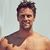# No One Can Pass This Basic Math Test From 1954

## In math, you're either right or you're wrong. Right?Created by Michael Rogers
On Apr 10, 2019

25% of 1,000 = ?

Which is equal to a quarter of its square?

41.1 - 40.3 = ?

100 x ½ = ?

19 x 19 = ?

24 ÷ 6 (8 – 4) = ?

What are the dimensions of a square whose perimeter and area are equal in value?

½ of ½ of ½ of ½ of 128 = ?

1000 x 1000 = ?

9 - 9 ÷ 9 + 9 - 9 ÷ 9 = ?

What is the square root of 225?

1110/10 = ?

½ ÷ ½ = ?

12 + 12 (12 – 12) = ?

120% = ?

NAILED IT!

NAILED IT!

You sure know your addition from your subtraction:) We gave this basic math test from 1954 to 300 students and only 4% passed it.

You think logically about mathematics. You visualize patterns, you make predictions, and you can come up with different ways to approach any given problem.

Being good at math gives you an advantage in life, and like most mathematicians you’re willing to take risks because you’re not intimidated by the unknown.

Amazing! You keep this up!

FAILED!

FAILED!

You totally blew it, but don’t worry too much about it. We gave this basic math test from 1954 to 300 students and only 4% passed.

Most pioneering scientists failed in high school. Use your logic and double-check your work, and who knows… you might be on your way to becoming a ground-breaking mathematician!

These are 10 of the World CRAZIEST Ice Cream Flavors
Created by Tal Garner
On Nov 18, 2021